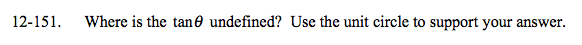### Home > A2C > Chapter 12 > Lesson 12.3.2 > Problem12-151

12-151.

Where is the tan θ undefined? Use the unit circle to support your answer. Homework Help ✎$\text{Remember tan\theta=\frac{sin\theta}{cos\theta}.}$

Where is cosθ = 0?